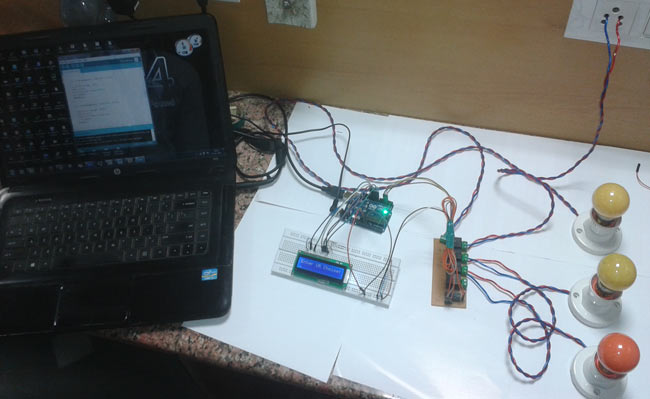# Variable Power Supply By Arduino Uno

In this tutorial we will develop a 5V variable voltage source from Arduino Uno. For that we are going use ADC (Analog to Digital Conversion) and PWM (Pulse Width Modulation) feature.

Some digital electronic modules like accelerometer work on voltage 3.3V and some work on 2.2V. Some even work on lower voltages. With this we cannot get a regulator for every one of them. So here we will make a simple circuit which will provide a voltage output from 0-5 volts at a resolution of 0.05V. So with this we may provide voltages accurately for the other modules.This circuit can provide currents up to 100mA, so we can use this power unit for most of the sensor modules without any trouble.  This circuit output can also be used to charge AA or AAA rechargeable batteries. With the display in place we can easily see the power fluctuations in the system. This variable power supply unit contains button interface for the voltage programming. The working and circuit is explained below.

Hardware: Arduino Uno, Power supply (5v), 100uF capacitor (2 pieces), button (2 pieces), 1KΩ resistor (3 pieces), 16*2 characters LCD, 2N2222 transistor.

Software: Atmel studio 6.2 or AURDINO nightly.

### Circuit Diagram and Working Explanation

The circuit for variable voltage unit using arduino is shown in below diagram.The voltage across output is not completely linear; it will be a noisy one. To filter out the noise capacitors are placed across output terminals as shown in figure. The two buttons here are for voltage increment and decrement. The display unit shows the voltage at the OUTPUT terminals.

Before going for working we need to look into ADC and PWM features of Arduino UNO.

Here we are going to take the voltage provided at the OUTPUT terminal and feed it into one of ADC channels of Arduino. After conversion we are going to take that DIGITAL value and we will relate it to voltage and show the result in 16*2 display. This value on display represents the variable voltage value.

ARDUINO has six ADC channels, as show in figure. In those any one or all of them can be used as inputs for analog voltage. The UNO ADC is of 10 bit resolution (so the integer values from (0-(2^10) 1023)).This means that it will map input voltages between 0 and 5 volts into integer values between 0 and 1023. So for every (5/1024= 4.9mV) per unit.

Here we are going to use A0 of UNO.

First of all the UNO ADC channels has a default reference value of 5V. This means we can give a maximum input voltage of 5V for ADC conversion at any input channel. Since some sensors provide voltages from 0-2.5V, with a 5V reference we get lesser accuracy, so we have a instruction that enables us to change this reference value.  So for changing the reference value we have (“analogReference();”) For now we leave it as.

As default we get the maximum board ADC resolution which is 10bits, this resolution can be changed by using instruction (“analogReadResolution(bits);”). This resolution change can come in handy for some cases. For now we leave it as.

Now if the above conditions are set to default, the we can read value from ADC of channel ‘0’ by directly calling function “analogRead(pin);”, here “pin” represents pin where we connected analog signal, in this case it would be “A0”.

Read More: Variable Power Supply By Arduino Uno

#### This Post / Project can also be found using search terms:

• variable power supply arduino
• power supply par arduino
• arduino power supply
• arduino power supply unit explanation